Published: 19 October 2019

# Level set medical image segmentation aided by cooperative quantum particle optimization with Lévy flights

Desheng Li1
Na Deng2
Xu Chen3
1College of Information and Network Engineering, Anhui Science and Technology University, Bengbu, 233000, China
2School of Computer, Hubei University of Technology, Wuhan, 430068, China
3School of Information and Safety Engineering, Zhongnan University of Economics and Law, Wuhan, 430073, China
Corresponding Author:
Desheng Li
Views 97

#### Abstract

Image segmentation plays an important part of image processing, and is also the premise and basis of image analysis and image understanding and recognition. Among the level set based methods, the original Local Binary Fitting (LBF) algorithm is a successful deterministic algorithm that suffers from sensitization to size of the local minimum, image contours, shapes, and initial positions. Among them, Level Set method promotes the two-dimensional problem to the three-dimensional one and then solves it using implicit method to express closed curve of plane. In this article, a novel Level Set model aided by PSO was proposed to solve automated medical image segmentation. The experimental result of segmentations on the benchmark shows that our proposed method is effective to both simple and complex medical images.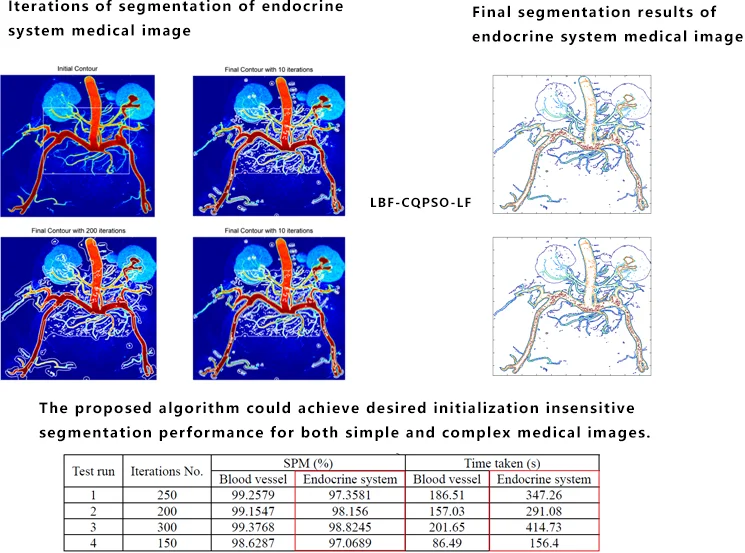#### Highlights

• The Gaussian filtering is used to smooth the level set rather than the penalty term
• As the import of the Lévy Flights, the noise disturbance is greatly reduced.
• The proposed algorithm could reach high SPM, i.e., achieve desired initialization insensitive segmentation performance for both simple and complex medical images.

## 1. Introduction

Image segmentation plays an important part of image processing, and is also the premise and basis of image analysis and image understanding and recognition. As the split structure is surrounded by other ones with similar structural strength, many existing classic segmentation techniques  (multi-threshold technology, regional growth and morphological filtering, etc.) just get general effect of segmentation.

Among them, Level Set method  promotes the development of the initiative geometric active contour model greatly as the mainstream direction of the methods based on energy functional theory. However, there are also some problems and/or shortcomings: for example, it requires the whole updates for the level set function values of all points of the entire image in the topology changes of adaptive evolution curve, which consume a lot of calculation; numerical convolution and partial differential equations also spend abundant computing time. Therefore, the overall efficiency of level set method is not high and difficult to be applied into practical applications. Especially, some level set methods, such as LBF method [3, 4], are sensitive to size of the local image contours, shapes, and initial positions. In addition, the most current level set models are usually non-convex energy functionals, whose solutions are the local minima rather than global ones. So it is difficult to achieve the desired segmentation results, but also affects the effectiveness of the algorithm.

In recent years, with the search ability, fast convergence speed and other characteristics, particle swarm optimization has been becoming the in-fact standard optimization algorithm. One important stream of research on particle swarm itself includes multi-population co-evolution, space partition and contraction. One most common and effective type is the cooperative particle swarm algorithm (Cooperarive PSO, CPSO) proposed by Fans Van den Bergh , which starts at the partition on the dimension of the particles of standard particle swarm optimization, and lets the multiple groups optimize respectively, and then calculates the fitness totally and updates by rules. In our previous work [6, 7], we developed an algorithm combining the Lévy flights and dynamic areas and then applied it in the realistic problems.

The current study shows that the particle swarm optimization has not been deeply embedded in the level set method as an organic integrity. It is possible to use PSO to replace some unnecessary convolution operations and take the advantages of strong searching capability and fast convergence speed. Moreover, most research also not combine the regularization model into it and promote the global performance. On the other side, the image segmentation algorithm based on level set is essentially an optimization problem, which minimizing the energy functional. Hence, the variational level set model of energy functional minimization problem could be formalized into meta-heuristic optimization problem, and by using the particle swarm optimization method and level set competitive image segmentation method. In this article, we embed the particle swarm optimization into the LBF model and algorithm to implement the inner optimization operation and test it on the medical image segmentation.

## 2. Review of LBF algorithm

### 2.1. The classic LBF algorithm

In LBF, the complete curve evolution equation is as follows:

1
$\frac{\partial \varphi }{\partial t}=\mu \left(∆\varphi -div\left(\frac{\nabla \varphi }{\left|\nabla \varphi \right|}\right)\right)+\nu {\delta }_{ϵ}\left(\varphi \right)div\left(\frac{\nabla \varphi }{\left|\nabla \varphi \right|}\right)-{\delta }_{ϵ}\left(\varphi \right)\left({\lambda }_{1}{e}_{1}-{\lambda }_{2}{e}_{2}\right),$

where ${e}_{1}\left(\bullet \right)$ and ${e}_{2}\left(\bullet \right)$ are defined as follows:

2
${e}_{1}\left(x\right)={\int }_{\mathrm{\Omega }}^{}{K}_{\sigma }\left(x-y\right){\left|I\left(y\right)-{f}_{1}\left(x\right)\right|}^{2}dy,{e}_{2}\left(x\right)={\int }_{\mathrm{\Omega }}^{}{K}_{\sigma }\left(x-y\right){\left|I\left(y\right)-{f}_{2}\left(x\right)\right|}^{2}dy.$

Heaviside function $H$ is approximated by a smooth function ${H}_{ϵ}$ which is defined by the following formula:

3
${H}_{ϵ}\left(x\right)=\frac{1}{2}\left[1+\frac{2}{\pi }\mathrm{a}\mathrm{r}\mathrm{c}\mathrm{t}\mathrm{a}\mathrm{n}\left(\frac{x}{ϵ}\right)\right].$

The fitting functions ${f}_{1}\left(x\right)$ and ${f}_{2}\left(x\right)$ will be updated according to the following equations:

4
${f}_{1}\left(x\right)=\frac{{K}_{\sigma }\left(x\right)*\left[{H}_{ϵ}\left(\varphi \right)I\left(x\right)\right]}{{K}_{\sigma }\left(x\right)*{H}_{ϵ}\left(\varphi \right)}{,f}_{2}\left(x\right)=\frac{{K}_{\sigma }\left(x\right)*\left[1-{H}_{ϵ}\left(\varphi \right)I\left(x\right)\right]}{{K}_{\sigma }\left(x\right)*\left[1-{H}_{ϵ}\left(\varphi \right)\right]},$

where $ϵ$ is a positive constant which is often set to 1.0 to equal to the fixed space steps $\delta \left(\bullet \right)$ is the Dirac function and the corresponding derivative of ${H}_{ϵ}$ could be the smoothed Dirac function with the below form:

5
${\delta }_{ϵ}\left(\varphi \right)={H}_{ϵ}^{\text{'}}\left(x\right)=\frac{d}{dx}{H}_{ϵ}\left(x\right)=\frac{1}{\pi }\frac{ϵ}{{ϵ}^{2}+{x}^{2}}.$

The main procedure of classic LBF can be summarized as following Algorithm 1 (Table 1). Firstly, the initial level set function ${\varphi }^{0}$ is simply defined as a binary function:

6
${\varphi }^{0}\left(x\right)=\left\{\begin{array}{c}-{c}_{0},x\in {R}^{2},\\ {c}_{0},\text{e}\text{l}\text{s}\text{e},\end{array}\right\$

where ${c}_{0}$ is a constant and ${R}^{2}$ is an arbitrarily given subset in the image domain.

Table 1Algorithm 1

 Algorithm 1. The pseudo-code of LBFInitialization: Read the input image $I:\Omega \subset {R}^{2}$.Build the initial level set function ${\varphi }^{0}$.Initialize the iteration number $n=0$.Scale parameter in Gaussian kernel.Repeat:Compute Heaviside function according to Eq. (3);Compute Dirac function according to Eq. (5);Compute ${e}_{i}$ according to Eq. (2);Update the value of ${f}_{1}\left(x\right)$ and ${f}_{2}\left(x\right)$ using Eq. (4);Update the level set function as ${\varphi }^{n+1}$ according to Eq. (1);Until $\left|{\varphi }^{n+1}-{\varphi }^{n}\right|;Output the segmentation result ${\varphi =\varphi }^{n+1}$.

## 3. Our proposed algorithms

### 3.1. CQPSO-LF algorithm

Based on our previous work in [6, 7], we have presented the proposed CQPSO-LF algorithm in steps in Algorithm 2 (Table 2).

Table 2Algorithm 2

 Algorithm 2. The pseudo-code of CQPSO-LFInitialization: Generate the positions randomly.RepeatSubSwarm Evaluation: Evaluate the fitness values of particles in sub-swarms according to the fitness function, and get ${C}_{d}$, ${P}_{id}^{best}$, and ${P}_{gd}^{best}$.SubSwarm Disturbance: Obtain the values ${P}_{gd}^{best\text{'}}$, ${C}_{d}^{\text{'}}$, by Lévy flights disturbance.Overall Evaluation: Elect the compositional global best position ${P}_{cgd}^{best}$.Overall Disturbance: Obtain the ${P}_{cgd}^{best\text{'}}$ by Lévy flights disturbance.Update Position: Renovate the positions of particles ${P}_{id}^{t+1}$.Until iteration > TH.

### 3.2. CQPSO-LF aided LBF algorithm (LBF-CQPSO-LF)

The original LBF algorithm is a deterministic algorithm that is also sensitive to size of the local image contours, shapes, and initial positions. In light of this shortcoming of the algorithm, we propose a new hybrid model in this article to utilize a population based swarm intelligence algorithm to select the good candidate contours with the global minimum of the fitting energy functional. Meanwhile, the level set method is also used to evolve the candidate contours and also get the cost function. During the iterations, the initial seeds are elected by the CQPSO-LF algorithm to achieve the best performance segmentation of the image. The whole framework of the CQPSO-LF aided LBF Algorithm (LBF-CQPSO-LF) is described in the Algorithm 3 (Table 3).

## 4. Experimental results and analysis

The aim of the experiment is to evaluate the effectiveness of LBF-CQPSO-LF method. At first, we choose one simple blood vessel image to test the validity of the method. The 3D landscape of the blood vessel image is shown in Fig. 1. As shown in Fig. 2, the method could not only segment out the desired objects increasingly, but also is stable to initial contours. Meanwhile, the preprocessing of the image is considered to make the level set regularized as much as possible. To achieve this purpose, we use the Gaussian filtering process to smooth the level set rather than the penalty term proposed by Li et al. [3, 4], which has been verified as a good substitute in literature [10, 11].

Table 3Algorithm 3

 Algorithm 3. The pseudo-code of LBF-CQPSO-LFInitialization: Read the input image $I:\mathrm{}\mathrm{\Omega }\subset {\mathrm{R}}^{2}$.Build the initial level set function ${\varphi }^{0}$.Initialize the iteration number $n=0$.Scale parameter in Gaussian kernel.While iteration < THFor $\mathbit{k}=\mathbf{}$1 to $N$Compute Heaviside function according to Eq. (3);Compute Dirac function according to Eq. (5);Compute ${e}_{i}$ according to Eq. (2);Upate the value of ${f}_{1}\left(x\right)$ and ${f}_{2}\left(x\right)$ using Eq. (4);Upate the level set function as ${\varphi }^{n+1}$ according to Eq. (1);Until$\left|{\varphi }^{n+1}-{\varphi }^{n}\right|;Output the segmentation result ${\varphi =\varphi }^{n+1}$.End ForFor$\mathbit{k}=\mathbf{}$1 to $N$SubSwarm Evaluation: Evaluate the fitness values ${E}^{LBF}\left(C,{f}_{1},{f}_{2}\right)$ of particles in sub-swarms according to the fitness function, and get ${C}_{d}$, ${P}_{id}^{best}$, and ${P}_{gd}^{best}$.SubSwarm Disturbance: Obtain the values ${P}_{gd}^{best\text{'}}$, ${C}_{d}^{\text{'}}$, by Lévy flights disturbance.Overall Evaluation: Elect the compositional global best position ${P}_{cgd}^{best}$.Overall Disturbance: Obtain the ${P}_{cgd}^{best\text{'}}$ by Lévy flights disturbance.Update Position: Renovate the positions of particles ${P}_{id}^{t+1}$.End ForEnd While

Fig. 13D landscape of the blood vessel image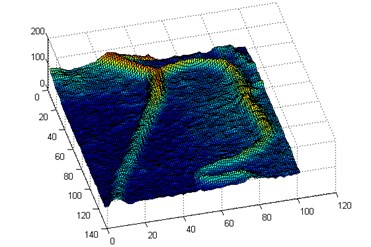In the sequent experiment, we utilized the LBF-CQPSO-LF in the real application scenario, i.e., an endocrine system medical image. To show the details explicitly, we transformed the image into pseudo-color in the view of Matlab. The initial contour and ones in the iterations are as shown in Fig. 3. The initial rectangular region is fixed in the center of the image, which is not sensitive to the final result any more. Due to the stochastic characteristic of this algorithm, some targets with weak boundaries could be well identified at Fig. 3(b-d).

The final segmentation results of endocrine system medical image after post-processing can be found in Fig. 4(a, b). Totally, the proposed algorithm can avoid the trapping in the local minima when the energy functional evolves. Moreover, as the import of the Lévy Flights, the noise disturbance is greatly reduced. Especially, after removing trivial edges, the refined segmentation can be seen in the Fig. 4(b) with the integrated and clean topological structures, which could be the input of the further analysis.

Fig. 2Iterations of segmentation of blood vessel image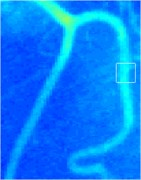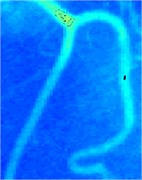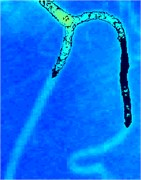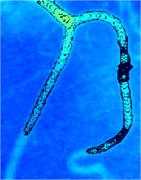Fig. 3Iterations of segmentation of endocrine system medical image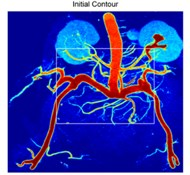a)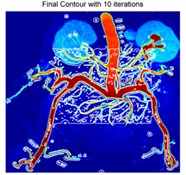b)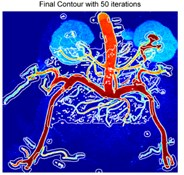c)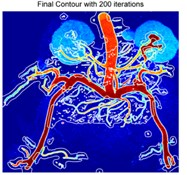d)

Fig. 4Final segmentation results of endocrine system medical image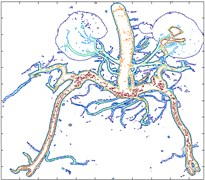a)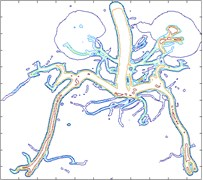b)

Table 4Performance of LBF-CQPSO-LF

 Test run Iterations No. SPM (%) Time taken (s) Blood vessel Endocrine system Blood vessel Endocrine system 1 250 99.2579 97.3581 186.51 347.26 2 200 99.1547 98.156 157.03 291.08 3 300 99.3768 98.8245 201.65 414.73 4 150 98.6287 97.0689 86.49 156.4

To evaluate the performance of proposed algorithm, LBF-CQPSO-LF, we adopted an index called Segmentation Performance Measure (SPM) imported in literature  as an benchmark, where the Automatically Segmented Image (ASI) is used to compare with the Manually Segmented Image (MSI) to calculate the similarity by Eq. (7). Table 4 presents the quantitative segmentation performance of blood vessel and endocrine system sample images by SPM and running time. It can be seen that the proposed algorithm could reach high SPM, i.e., achieve desired initialization insensitive segmentation performance for both simple and complex medical images:

7
$SPM=\frac{2*\left(ASI\cap MSI\right)}{\left|ASI\right|+\left|MSI\right|}.$

## 5. Conclusions

In this article, a novel level set model aided by PSO was proposed to solve automated medical image segmentation. In our algorithm, the variational level set model of energy functional minimization problem has been formalized into the meta-heuristic optimization problem. Hence, we embed the particle swarm optimization with Lévy flights into the classic LBF to implement the inner optimization operation in order to accelerate convergence. According to the state of art, our method is novel and unique in this research field of world. The experimental result of segmentations on the benchmark shows that our proposed method is effective to both simple and complex medical images. As our future studies, we will investigate how to expand this method to the three-dimension case and consider multi-phase level sets circumstance. Moreover, as the limitation of slow convergence, we aim to promote the rate of convergence according to some approximate methods. Additionally, we will also explore more effective models to evolution of the level set curves of medical images according to their characteristics.

21 September 2019
Accepted
28 September 2019
Published
19 October 2019
SUBJECTS
Biomechanics and biomedical engineering
Keywords
level set
segmentation
particle optimization
Lévy flights
active contour
Acknowledgements

This research was supported by Natural Science Foundation of Anhui Province under Grant 1708085MF161, in part by Key Project of Natural Science Research of Universities in Anhui under Grant KJ2015A236, Philosophical and Social Sciences Research Project of Hubei Education Department under Grant 19Q054, and Research Foundation for Advanced Talents of the Hubei University of Technology under Grant BSQD12131.